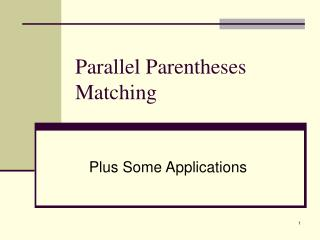Download PresentationParallel Parentheses Matching

# Parallel Parentheses Matching - PowerPoint PPT PresentationDownload Presentation## Parallel Parentheses Matching

- - - - - - - - - - - - - - - - - - - - - - - - - - - E N D - - - - - - - - - - - - - - - - - - - - - - - - - - -
##### Presentation Transcript

1. Parallel Parentheses Matching Plus Some Applications

2. Parentheses Matching Problem Definition: • Given a well-formed sequence of parentheses stored in an array, determine the index of the mate of each parentheses stored in the array

3. Example

4. Sequential Solution • Traditional solution uses a stack • Push left parentheses, pop for a right parenthesis; these are a pair • Can this method be implemented in parallel? Why or why not?

5. Parallel Solution: Divide & Conquer • Lemma 1: The mate of a parenthesis at an odd position in a balanced string lies in an even position (and vice versa). • Lemma 2: If a balanced string has no left parenthesis at an even location (or, equivalently, a right at an odd location), then the mate of each left parenthesis in the string lies immediately to its right.

6. Lemma 2 • Any string that satisfies Lemma 2 is of form ( ) ( ) ( ) ( )….( ) and is referred to as form F.

7. Algorithm Overview • Each left position at an odd position and each right position are marked with a 0. • All others are marked with a 1. These 2 disjoint sets are copied (packed) into a new array. • Repeat for the 2 sets. • Stop when each new substring is of form F.

8. Algorithm Match For i = 1 to log n – 1 do if “(“ & index is odd then mark 0 else mark 1 Use segmented prefix sums to compute new index for each parenthesis Move parentheses to new location Determine if string is now in form F; if not, terminate – unbalanced string Match parentheses and store in original array

9. Example

10. Example – Keep Index

11. Segmented Prefix Sum Problem Definition Given an array containing elements, some marked 0 and some marked 1. Compute the prefix sum of each subset. (For this application the sums will be on values of 1, to number the items.)

12. Segmented Prefix Sum - Example How can this be accomplished with one prefix sums operation?

13. Parentheses Matching on Hypercube • Use the Divide & Conquer strategy • Consider 2 processors • Each PC – assign 0/1 • P0 send 1’s to P1; P1 send 0’s to P0 • Each solve the sub-problem • Does the problem split evenly? • Consider Large problem – P0 & P2 take 0 items, P1 & P3 take 1 items

14. PPM - Hypercube • Overview of Algorithm • 2-Cube: Special case of 2 pc hypercube • 4-Cube: Used to partition large sub-problems consisting of 4 pc cubes • Match: The Driving Algorithm

15. Data Distribution • INPUT array is divided into P equal partitions of size n/p • First n/p items are given to P0, next n/p items to P1, etc. • Final MATCH information for each item is stored in the original PC

16. Data Distribution • Array consists of elements INPUT which holds the parentheses & MATCH which will hold the final matching information • Local Match: if the match is determined by the pc in which the match information is to be stored • Non-local: otherwise

17. Algorithm 2-Cube • Mark left and right parentheses with 0/1 as previously discussed • P0 & P1 exchange entries – P0 contains 0 and P1 contains 1 • Each PC use stack to sequentially match parentheses • Send non-local match operation to appropriate processor

18. Algorithm 2-Cube – Step 1 Mark 0/1 P0 P1

19. Algorithm 2-Cube – Step 2 & 3 Exchange & Match P0 P1

20. Algorithm 2-Cube – Step 4 Send non-local match information P0 P1

21. Algorithm 4-Cube • Basis of Algorithm Match • Insures near-equal data distribution • Overview • Phase 1: local matches determined sequentially & communicated • Phase 2: Unmatched parentheses marked, redistributed; P0 & P1 have 0’s, P2 & P3 have 1’s (half each)

22. Algorithm 4- Cube Phase 1: Sequential Processing 1. Each PC use stack to match 2. Send non-local Match to other PC 3. Count unmatched parentheses; prefix sum to reindex

23. Algorithm 4-Cube Phase 2 1. Mark parentheses with 1/0 2. P0 & P2 exchange: P0=0 & P2=1 Likewise, P1=0 and P3=1 • P0 & P1 exchange number information; likewise for P2 & P3 • P0 & P1 exchange entries; P0 obtains 1st half: likewise for P2 & P3

24. Algorithm 4-Cube Distribution of Data – 64 entries

25. Algorithm Hypercube Match • Each subcube of size 4 executes 4-Cube // Logically P/2 subcubes with independent subproblems • Each subcube (p/2) prefix sums to determine new index • Each subcube from Step 1 recursively repeat 1, 2, 3 until each subcube is of size 2 4. Execute 2-Cube to complete the solution

26. Algorithm Hypercube Match Complexity Analysis • O (log 2 p + n/p log p) • For p=n/log n simplifies to O(log 2 n) Is the Algorithm Optimal?